407 493 6601

# Precalculus - Parabolas

## Introduction

• Parabola is the set of all points in a plane equidistant from a fixed point (focus) and a line (directrix).
• Parabola is formed when the intersecting plane to a right circular cone is parallel to the slant height of the cone.
• The eccentricity of a parabola is equal to 1.
• The axis of symmetry is given by $y=k$ when the parabola opens to the right or opens to the left.
• The axis of symmetry is given by $x=h$ when the parabola opens upward or downward.
• The parabola ${\mathbf{\left(}\mathbf{x}\mathbf{-}\mathbf{h}\mathbf{\right)}}^{\mathbf{2}}\mathbf{=}\mathbf{4}\mathbit{c}\mathbf{\left(}\mathbf{y}\mathbf{-}\mathbf{k}\mathbf{\right)}$ opens upward when $c>0$ and downward when $c<0.$
• The parabola ${\mathbf{\left(}\mathbf{y}\mathbf{-}\mathbf{k}\mathbf{\right)}}^{\mathbf{2}}\mathbf{=}\mathbf{4}\mathbit{c}\mathbf{\left(}\mathbf{x}\mathbf{-}\mathbf{h}\mathbf{\right)}$ opens to the right when $c>0$ and opens to the left when $c<0.$
• The general equations of the parabola are as follows:

${\mathbit{y}}^{\mathbf{2}}\mathbf{+}\mathbit{D}\mathbit{x}\mathbf{+}\mathbit{E}\mathbit{y}\mathbf{+}\mathbit{F}\mathbf{=}\mathbf{0}$ when the axis of symmetry is parallel to the x-axis.

${\mathbit{x}}^{\mathbf{2}}\mathbf{+}\mathbit{D}\mathbit{x}\mathbf{+}\mathbit{E}\mathbit{y}\mathbf{+}\mathbit{F}\mathbf{=}\mathbf{0}$ when the axis of symmetry is parallel to the y-axis.

• In other references, it is important to note the following general equations of the parabola: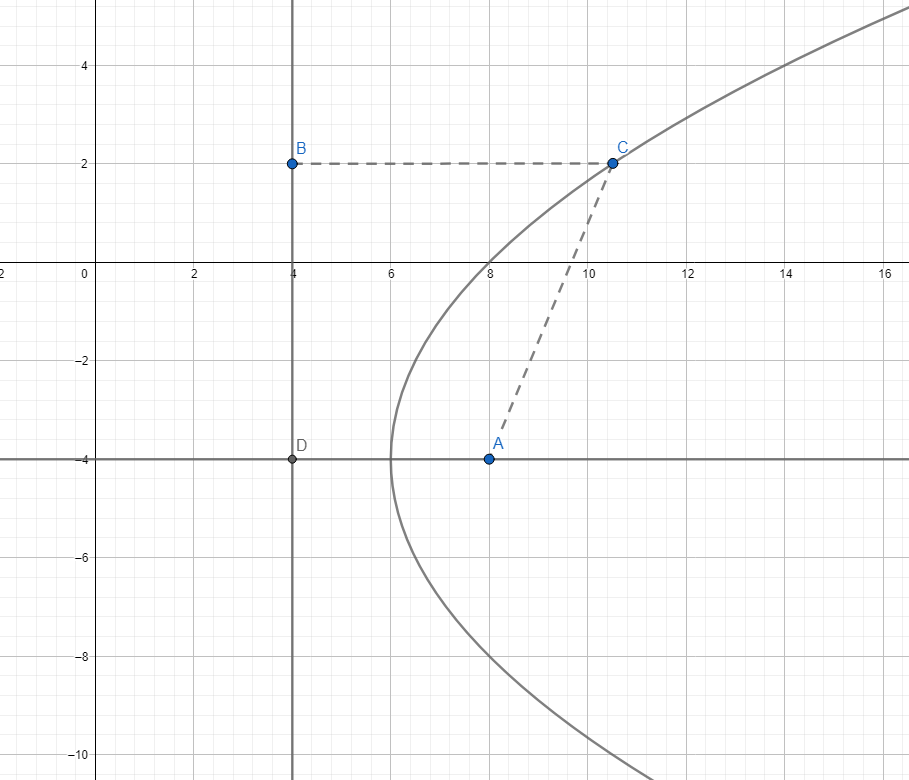• No matter where we put point C in the parabola, as shown above, the distances between Focus A and point C and between point C and point B are always equal. The line BD is the directrix of the parabola.

## Basic Concepts: Standard Equations and Graphs of the Parabola with vertex at (0,0)

The standard equations of the parabola with center at $\left(0,0\right)$ are described below with their corresponding graphs:

1. ${\mathbit{y}}^{\mathbf{2}}\mathbf{=}\mathbf{4}\mathbit{c}\mathbit{x}$, vertex at $\left(0,0\right)$

This is the standard equation of the parabola that opens to the right.

The graph of is shown below.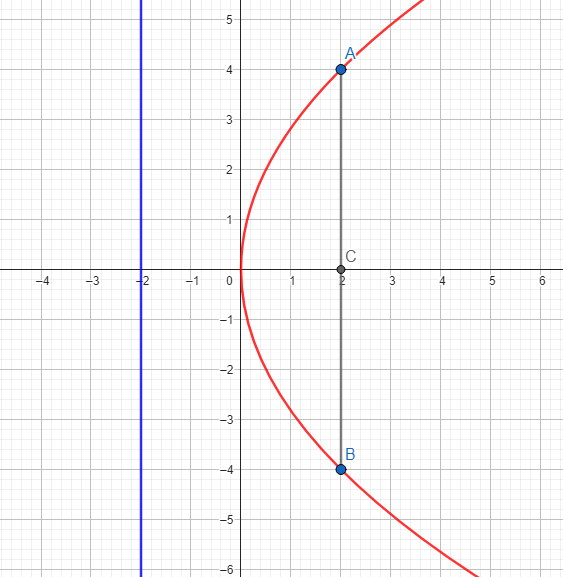Directrix is $x=-2$

Focus:

Endpoints of the Latus Rectum:

and ${L}_{2}\left(c,-2c\right)=B\left(2,-4\right)$

2. ${\mathbit{y}}^{\mathbf{2}}\mathbf{=}\mathbf{-}\mathbf{4}\mathbit{c}\mathbit{x}$, vertex at $\left(0,0\right)$

This is the standard equation of the parabola that opens to the left.

The graph of is shown below.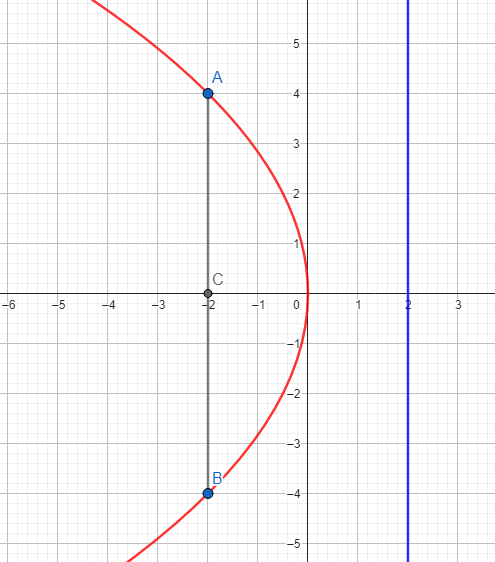Directrix is $x=2$

Focus:

Endpoints of the Latus Rectum:

and ${L}_{2}\left(-c,-2c\right)=B\left(-2,-4\right)$

3. ${\mathbit{x}}^{\mathbf{2}}\mathbf{=}\mathbf{4}\mathbit{c}\mathbit{y}$, vertex at $\left(0,0\right)$

This is the standard equation of the parabola that opens upward.

The graph of ${x}^{2}=8y$ is shown below.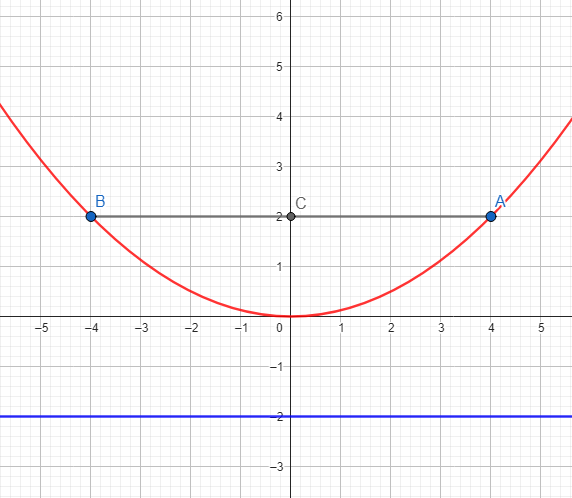Directrix is $y=-2$

Focus:

Endpoints of the Latus Rectum:

and

4. ${\mathbit{x}}^{\mathbf{2}}\mathbf{=}\mathbf{-}\mathbf{4}\mathbit{c}\mathbit{y}$, vertex at $\left(0,0\right)$

This is the standard equation of the parabola that opens downward.

The graph of ${x}^{2}=-8y$ is shown below.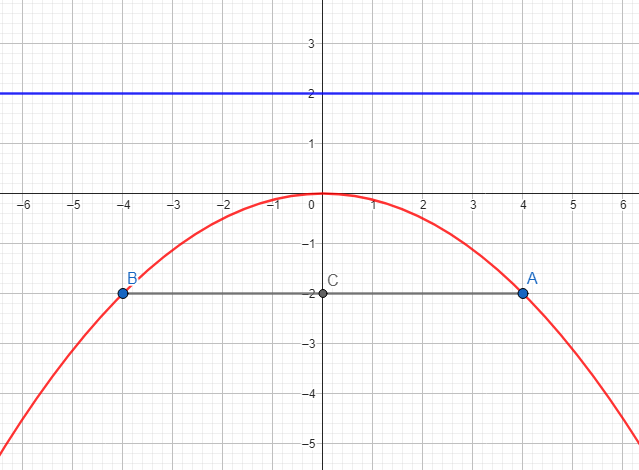Directrix is $y=2$

Focus: $F\left(0,-c\right)=C\left(0,-2\right)$

Endpoints of the Latus Rectum:

${L}_{1}\left(2c,-c\right)=A\left(4,-2\right)$ and ${L}_{2}\left(-2c,-c\right)=B\left(-4,-2\right)$

## Basic Concepts: Standard Equations and Graphs of the Parabola with vertex at (h,k)

The standard equations of the parabola with vertex at $\left(h,k\right)$ with their corresponding graphs are briefly discussed.

1. ${\mathbf{\left(}\mathbf{y}\mathbf{-}\mathbf{k}\mathbf{\right)}}^{\mathbf{2}}\mathbf{=}\mathbf{4}\mathbit{c}\mathbf{\left(}\mathbf{x}\mathbf{-}\mathbf{h}\mathbf{\right)}\mathbf{,}$ vertex at $\left(h,k\right)$

This standard equation represents a parabola that opens to the right.

The graph of ${\left(y-2\right)}^{2}=8\left(x-3\right)$ is shown below.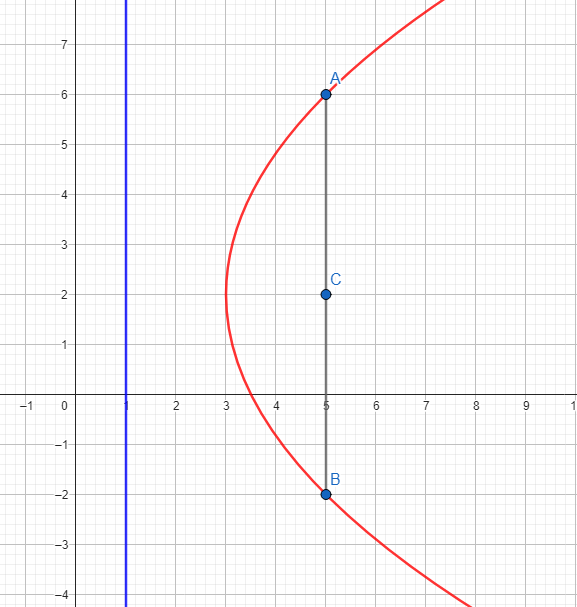Vertex

Equation of the Directrix:

$x=h-c\to x=3-2\to x=1$

Focus:

Endpoints of the Latus Rectum:

2. vertex at $\left(h,k\right)$

This standard equation represents a parabola that opens to the left.

The graph of ${\left(y-2\right)}^{2}=-8\left(x-3\right)$ is shown below.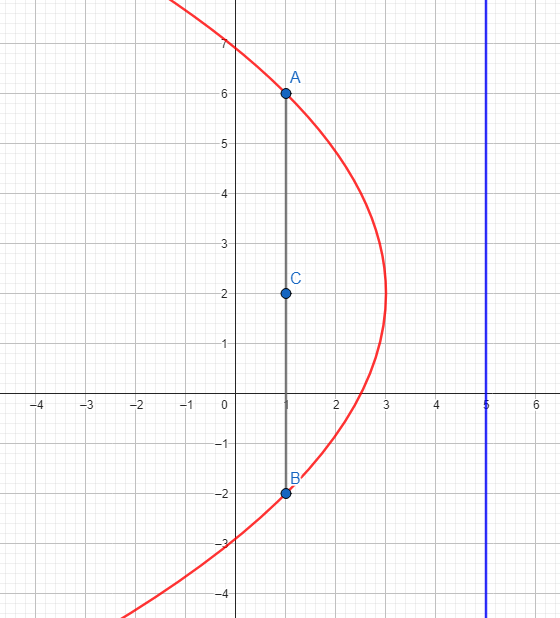Vertex

Equation of the DIrectrix:

$x=h-c\to x=3-\left(-2\right)\to x=5$

Focus:

Endpoints of the Latus Rectum:

3. vertex at $\left(h,k\right)$

This standard equation represents a parabola that opens upward

The graph of ${\left(x-3\right)}^{2}=8\left(y-4\right)$ is shown below.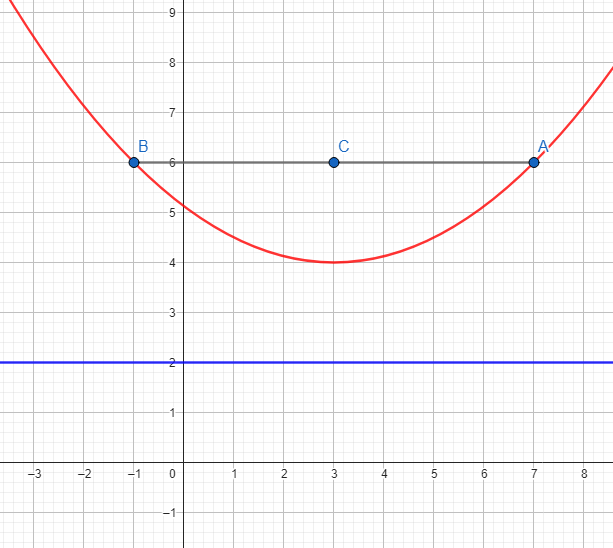Vertex

Equation of the Directrix:

$y=k-c\to y=4-c\to y=2$

Focus:

Endpoints of the Latus Rectum:

${L}_{2}\left(h-2c,k+c\right)=B\left(-1,6\right)$

4. vertex at $\left(h,k\right)$

This standard equation represents a parabola that opens downward.

The graph of ${\left(x-3\right)}^{2}=-8\left(y-4\right)$ is shown below.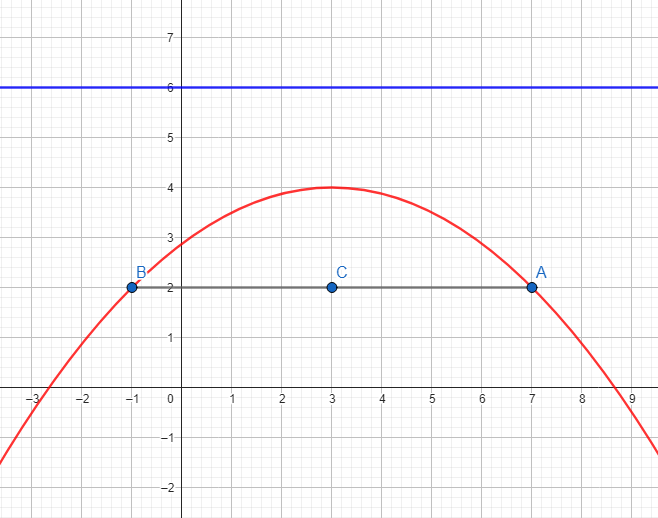Vertex

Equation of the Directrix:

$y=k-c\to y=4-\left(-2\right)\to y=6$

Focus:

Endpoints of the Latus Rectum:

## Solved Examples

Example 1. Determine the opening, coordinates of the vertex, coordinates of the focus, endpoints, and length of the latus rectum, and equation of the directrix of the parabola ${\mathbit{x}}^{\mathbf{2}}\mathbf{=}\mathbf{25}\mathbit{y}$.

Solution:

The parabola ${x}^{2}=25y$ is in the form of ${x}^{2}=4cy$.

The graph of this parabola opens upward since $c>0$.

The vertex is at the origin $\left(0,0\right)$.

The coordinates of the focus follow the form $F\left(0,c\right)$. In this case, we solve for $c$

Hence, the focus is at $\left(0,6\frac{1}{4}\right)$.

To solve for the endpoints of the latus rectum, we follow the form

Hence, the endpoints of the latus rectum are .

The length of the latus rectum is given by $L=4c$

In this case, we have:

Since this parabola is in the form of ${x}^{2}=4cy$, the equation of the directrix follows the form $y=-c$.

Equation of the Directrix: $y=-6\frac{1}{4}$

Example 2. Determine the opening, coordinates of the vertex, coordinates of the focus, endpoints, and length of the latus rectum, and the equation of the directrix of the parabola ${\left(y+3\right)}^{2}=-9\left(x-7\right)$.

Solution:

The parabola ${\left(y+3\right)}^{2}=-9\left(x-7\right)$ is in the form of ${\left(y-k\right)}^{2}=-4c\left(x-h\right)$

This parabola opens to the left since $c<0$ and its vertex is at $\left(7,-3\right)$

The coordinates of the focus of this parabola follows .

To solve for $c$, we have:

In solving for the coordinates of the focus and the endpoints of the latus rectum, disregard the sign of $c$.

The focus is $\left(4\frac{3}{4},-3\right)$.

The endpoints of the latus rectum are solved using the form .

In this example, the endpoints of the latus rectum are .

The length of the latus rectum is

The equation of the directrix is $x=h-c\to x=7-\left(-\frac{9}{4}\right)\to x=\frac{37}{4}$

## Cheat Sheet

 Standard Forms of a Parabola Coordinates Axis of Symmetry Equation of the Directrix Vertex Focus Latus Rectum ${y}^{2}=4cx$ $\left(0,0\right)$ $\left(c,0\right)$ $\left(c,±2c\right)$ $y=0$ $x=-c$ ${x}^{2}=4cy$ $\left(0,0\right)$ $\left(0,c\right)$ $x=0$ $y=c$ ${\left(y-k\right)}^{2}=4c\left(x-h\right)$ $\left(h,k\right)$ $\left(h±c,k\right)$ $\left(h±c,k±2c\right)$ $y=k$ $x=h-c$ ${\left(x-h\right)}^{2}=4c\left(y-k\right)$ $\left(h,k\right)$ $\left(h,k±c\right)$ $x=h$ $y=k-c$

## Blunder Areas

• Always inspect the sign of to know the opening of the parabola, the correct coordinates of the vertex, and other properties.
• In solving for the coordinates of the latus rectum and focus, neglect the sign of $c$.
• In solving for the equation of the directrix, always consider the sign of $c$.
• Parabolas in the form of are not representations of quadratic functions.
• Use the algebraic method in transforming the equation of the parabola in in vertex form (standard form). This is done using completing the square.
• The algebraic method and graphical method can both be used to determine, study, and interpret the characteristics/properties of a parabola.